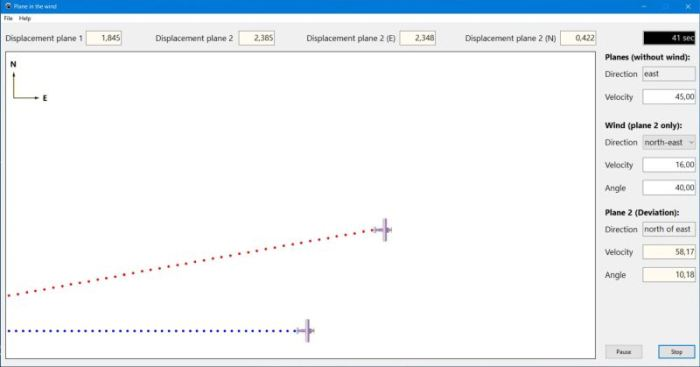# Computing: Free Pascal Programming

 Programming Home Home Contact

## Plane in the wind simulation (Plane).

Description: Kinematics related application, simulating a plane moving in the wind. Physicly spoken, it's a case of velocity addition, mathematically spoken a case of vector calculation. Just fill in the plane velocity (= velocity relative to the air mass) and the velocity and angle of the wind and push the "Start" button. The program calculates the plane total velocity and the angle it is deviated (from its original east-direction towards the north) and moves the two planes (plane 1 = no wind) accordingly.

Wind with north-east direction (angle = 0 - 90º) is tail-wind, thus the velocity of plane 2 is increased; wind with north-west direction (angle = 90º - 180º) is head wind, thus the velocity of the plane is decreased. Special cases: angle = 0: full tail-wind (from the west); angle = 90º: full cross-wind (from the south); angle = 180º: full head wind (from the east). The deviation angle depends on the relative velocities of the plane and the wind. Note that if this angle = 45º, the displacement of the plane towards the north (y-axis) is the same as its displacement towards the east (x-axis); this corresponds mathematically to sin(45º) = cos(45º).

Free Pascal features: Creating objects during program execution. Using a timer and images' "Left" and "Top" properties to create simple graphical animations. Using small shapes to "draw" curves.

Screenshot: